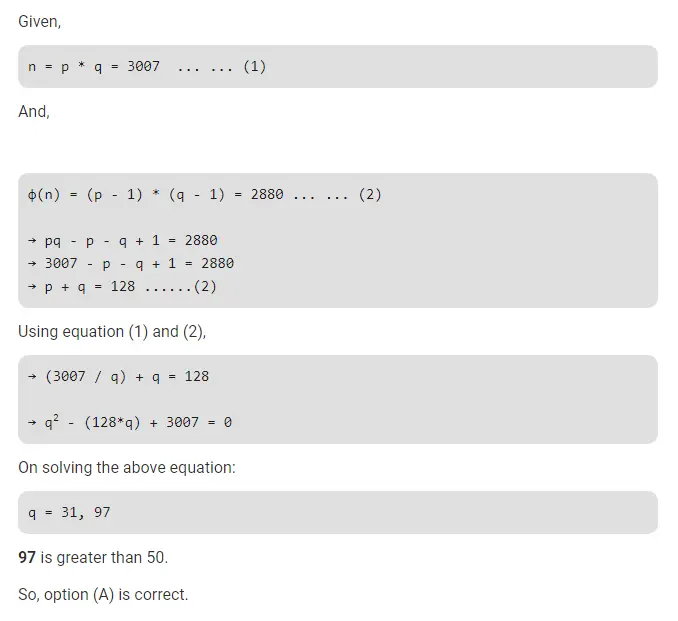# Gate CS-2019 Question Paper With Solutions

Q. 60 In an RSA cryptosystem, the value of the public modulus parameter n is 3007. If it is also is known that φ(n) = 2880, where φ( ) denotes Euler’s Totient Function, then the prime factors of n which is greater than 50 is _________ .

Note: This was Numerical Type question.

(A) 97

(B) 31

(C) 37

(D) 91